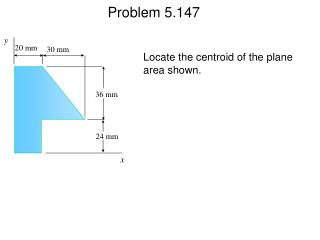Download Presentation20 mm

# 20 mm

Download Presentation## 20 mm

- - - - - - - - - - - - - - - - - - - - - - - - - - - E N D - - - - - - - - - - - - - - - - - - - - - - - - - - -
##### Presentation Transcript

1. Problem 5.147 y 20 mm 30 mm Locate the centroid of the plane area shown. 36 mm 24 mm x

2. y Problem 5.147 20 mm 30 mm Locate the centroid of the plane area shown. 36 mm 24 mm x 1. Decide how to construct the given area from common shapes. 2. Construct a table containing areas or length and the respective coordinates of the centroids. 3. When possible, use symmetry to help locate the centroid.

3. Problem 5.147 y 20 + 10 Decide how to construct the given area from common shapes. C1 C2 24 + 12 30 x 10 Dimensions in mm

4. Problem 5.147 Solution y 20 + 10 Construct a table containing areas and respective coordinates of the centroids. C1 C2 24 + 12 30 x 10 Dimensions in mm A, mm2 x, mm y, mm xA, mm3 yA, mm3 1 20 x 60 =1200 10 30 12,000 36,000 2 (1/2) x 30 x 36 =540 30 36 16,200 19,440 S 1740 28,200 55,440

5. Problem 5.147 y 20 + 10 XSA = S xA Then X (1740) = 28,200 X = 16.21 mm or C1 C2 YSA = S yA and 24 + 12 30 Y (1740) = 55,440 x 10 Y = 31.9 mm or Dimensions in mm A, mm2 x, mm y, mm xA, mm3 yA, mm3 1 20 x 60 =1200 10 30 12,000 36,000 2 (1/2) x 30 x 36 =540 30 36 16,200 19,440 S 1740 28,200 55,440

6. Problem 5.154 24 kN 30 kN a 0.3 m A B wA wB 1.8 m The beam AB supports two concentrated loads and rests on soil which exerts a linearly distributed upward load as shown. Determine (a) the distance a for which wA = 20 kN/m, (b) the corresponding value wB.

7. 24 kN 30 kN a 0.3 m A B wA wB 1.8 m Problem 5.154 The beam AB supports two concentrated loads and rests on soil which exerts a linearly distributed upward load as shown. Determine (a) the distance a for which wA = 20 kN/m, (b) the corresponding value wB. 1. Replace the distributed load by a single equivalent force. The magnitude of this force is equal to the area under the distributed load curve and its line of action passes through the centroid of the area. 2. When possible, complex distributed loads should be divided into common shape areas.

8. 24 kN 30 kN a 0.3 m C A B 20 kN/m wB 0.6 m 0.6 m RI RII 1 2 1 2 Problem 5.154 Replace the distributed load by a pair of equivalent forces. RI = (1.8 m)(20 kN/m) = 18 kN We have RII = (1.8 m)(wB kN/m) = 0.9 wB kN

9. + + Problem 5.154 24 kN 30 kN a 0.3 m C A B wB 0.6 m 0.6 m RII = 0.9 wB kN RI = 18 kN (a) SMC = 0: (1.2 - a)m x 24 kN - 0.6 m x 18 kN - 0.3m x 30 kN = 0 or a = 0.375 m (b) SFy = 0: -24 kN + 18 kN + (0.9 wB) kN - 30 kN= 0 or wB = 40 kN/m

10. Problem 5.157 y y = kx1/3 Locate the centroid of the volume obtained by rotating the shaded area about the x axis. a x h

11. y Problem 5.157 y = kx1/3 Locate the centroid of the volume obtained by rotating the shaded area about the x axis. a The procedure for locating the centroids of volumes by direct integration can be simplified: x h 1. When possible, use symmetry to help locate the centroid. 2. If possible, identify an element of volume dV which produces a single or double integral, which are easier to compute. 3. After setting up an expression for dV, integrate and determine the centroid.

12. Problem 5.157 Solution y Use symmetry to help locate the centroid. Symmetry implies x dx y = 0 z = 0 x z r Identify an element of volume dV which produces a single or double integral. y = kx1/3 Choose as the element of volume a disk or radius r and thickness dx. Then dV = p r2 dx xel = x

13. Problem 5.157 Solution y x Identify an element of volume dV which produces a single or double integral. dx dV = p r2 dx xel = x x z r r = kx1/3 so that Now dV = p k2 x2/3dx y = kx1/3 a = kh1/3 k = a/h1/3 or At x = h, y = a : a2 h2/3 dV = p x2/3dx Then

14. a2 h2/3 dV = p x2/3dx 3 5 3 5 5 8 x = h 3 8 = p a2h2 Problem 5.157 Solution y Integrate and determine the centroid. x dx a2 h2/3 h V = p x2/3dx x z r 0 a2 h2/3 h [ ] 3 5 = p x5/3 y = kx1/3 0 = p a2h a2 h2/3 a2 h2/3 3 8 h xel dV = x (p x2/3 dx) = p [ x8/3 ] Also 0 3 8 x ( p a2h) = p a2h2